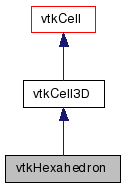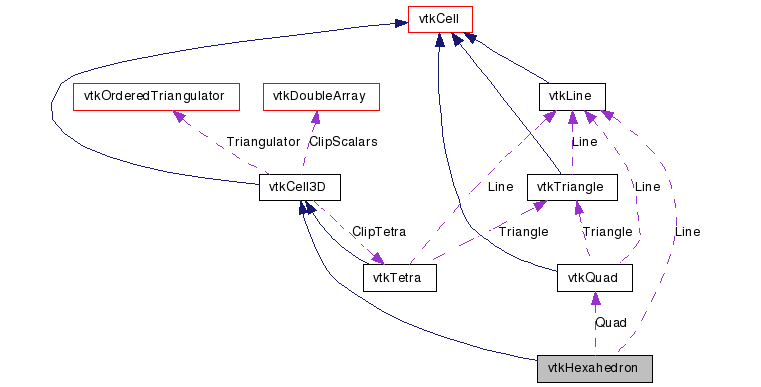# vtkHexahedron Class Reference

`#include <vtkHexahedron.h>`

Inheritance diagram for vtkHexahedron:[legend]
Collaboration diagram for vtkHexahedron:[legend]

## Detailed Description

a cell that represents a linear 3D hexahedron

vtkHexahedron is a concrete implementation of vtkCell to represent a linear, 3D rectangular hexahedron (e.g., "brick" topology). vtkHexahedron uses the standard isoparametric shape functions for a linear hexahedron. The hexahedron is defined by the eight points (0-7) where (0,1,2,3) is the base of the hexahedron which, using the right hand rule, forms a quadrilaterial whose normal points in the direction of the opposite face (4,5,6,7).

vtkConvexPointSet vtkPyramid vtkTetra vtkVoxel vtkWedge
Examples:
vtkHexahedron (Examples)
Tests:
vtkHexahedron (Tests)

Definition at line 45 of file vtkHexahedron.h.

static void InterpolationFunctions (double pcoords, double weights)
static void InterpolationDerivs (double pcoords, double derivs)
virtual void InterpolateFunctions (double pcoords, double weights)
virtual void InterpolateDerivs (double pcoords, double derivs)

## Public Types

typedef vtkCell3D Superclass

## Public Member Functions

virtual const char * GetClassName ()
virtual int IsA (const char *type)
void PrintSelf (ostream &os, vtkIndent indent)
int EvaluatePosition (double x, double *closestPoint, int &subId, double pcoords, double &dist2, double *weights)
void EvaluateLocation (int &subId, double pcoords, double x, double *weights)
int IntersectWithLine (double p1, double p2, double tol, double &t, double x, double pcoords, int &subId)
int Triangulate (int index, vtkIdList *ptIds, vtkPoints *pts)
void Derivatives (int subId, double pcoords, double *values, int dim, double *derivs)
virtual double * GetParametricCoords ()
void JacobianInverse (double pcoords, double **inverse, double derivs)
virtual void GetEdgePoints (int edgeId, int *&pts)
virtual void GetFacePoints (int faceId, int *&pts)
int GetCellType ()
int GetNumberOfEdges ()
int GetNumberOfFaces ()
vtkCellGetEdge (int edgeId)
vtkCellGetFace (int faceId)
int CellBoundary (int subId, double pcoords, vtkIdList *pts)
void Contour (double value, vtkDataArray *cellScalars, vtkIncrementalPointLocator *locator, vtkCellArray *verts, vtkCellArray *lines, vtkCellArray *polys, vtkPointData *inPd, vtkPointData *outPd, vtkCellData *inCd, vtkIdType cellId, vtkCellData *outCd)

## Static Public Member Functions

static vtkHexahedronNew ()
static int IsTypeOf (const char *type)
static vtkHexahedronSafeDownCast (vtkObject *o)
static int * GetEdgeArray (int edgeId)
static int * GetFaceArray (int faceId)

## Protected Member Functions

vtkHexahedron ()
~vtkHexahedron ()

vtkLineLine

## Member Typedef Documentation

 typedef vtkCell3D vtkHexahedron::Superclass

Reimplemented from vtkCell3D.

Definition at line 49 of file vtkHexahedron.h.

## Constructor & Destructor Documentation

 vtkHexahedron::vtkHexahedron ( ) ` [protected]`

 vtkHexahedron::~vtkHexahedron ( ) ` [protected]`

## Member Function Documentation

 static vtkHexahedron* vtkHexahedron::New ( ) ` [static]`

Create an object with Debug turned off, modified time initialized to zero, and reference counting on.

Reimplemented from vtkObject.

 virtual const char* vtkHexahedron::GetClassName ( ) ` [virtual]`

Reimplemented from vtkCell3D.

 static int vtkHexahedron::IsTypeOf ( const char * name ) ` [static]`

Return 1 if this class type is the same type of (or a subclass of) the named class. Returns 0 otherwise. This method works in combination with vtkTypeMacro found in vtkSetGet.h.

Reimplemented from vtkCell3D.

 virtual int vtkHexahedron::IsA ( const char * name ) ` [virtual]`

Return 1 if this class is the same type of (or a subclass of) the named class. Returns 0 otherwise. This method works in combination with vtkTypeMacro found in vtkSetGet.h.

Reimplemented from vtkCell3D.

 static vtkHexahedron* vtkHexahedron::SafeDownCast ( vtkObject * o ) ` [static]`

Reimplemented from vtkCell3D.

 void vtkHexahedron::PrintSelf ( ostream & os, vtkIndent indent ) ` [virtual]`

Methods invoked by print to print information about the object including superclasses. Typically not called by the user (use Print() instead) but used in the hierarchical print process to combine the output of several classes.

Reimplemented from vtkCell3D.

 virtual void vtkHexahedron::GetEdgePoints ( int edgeId, int *& pts ) ` [virtual]`

See vtkCell3D API for description of these methods.

Implements vtkCell3D.

 virtual void vtkHexahedron::GetFacePoints ( int faceId, int *& pts ) ` [virtual]`

See vtkCell3D API for description of these methods.

Implements vtkCell3D.

 int vtkHexahedron::GetCellType ( ) ` [inline, virtual]`

See the vtkCell API for descriptions of these methods.

Implements vtkCell.

Definition at line 60 of file vtkHexahedron.h.

 int vtkHexahedron::GetNumberOfEdges ( ) ` [inline, virtual]`

See the vtkCell API for descriptions of these methods.

Implements vtkCell.

Definition at line 61 of file vtkHexahedron.h.

 int vtkHexahedron::GetNumberOfFaces ( ) ` [inline, virtual]`

See the vtkCell API for descriptions of these methods.

Implements vtkCell.

Definition at line 62 of file vtkHexahedron.h.

 vtkCell* vtkHexahedron::GetEdge ( int edgeId ) ` [virtual]`

See the vtkCell API for descriptions of these methods.

Implements vtkCell.

 vtkCell* vtkHexahedron::GetFace ( int faceId ) ` [virtual]`

See the vtkCell API for descriptions of these methods.

Implements vtkCell.

 int vtkHexahedron::CellBoundary ( int subId, double pcoords, vtkIdList * pts ) ` [virtual]`

See the vtkCell API for descriptions of these methods.

Implements vtkCell.

 void vtkHexahedron::Contour ( double value, vtkDataArray * cellScalars, vtkIncrementalPointLocator * locator, vtkCellArray * verts, vtkCellArray * lines, vtkCellArray * polys, vtkPointData * inPd, vtkPointData * outPd, vtkCellData * inCd, vtkIdType cellId, vtkCellData * outCd ) ` [virtual]`

See the vtkCell API for descriptions of these methods.

Reimplemented from vtkCell3D.

 int vtkHexahedron::EvaluatePosition ( double x, double * closestPoint, int & subId, double pcoords, double & dist2, double * weights ) ` [virtual]`

Given a point x return inside(=1), outside(=0) cell, or (-1) computational problem encountered; evaluate parametric coordinates, sub-cell id (!=0 only if cell is composite), distance squared of point x to cell (in particular, the sub-cell indicated), closest point on cell to x (unless closestPoint is null, in which case, the closest point and dist2 are not found), and interpolation weights in cell. (The number of weights is equal to the number of points defining the cell). Note: on rare occasions a -1 is returned from the method. This means that numerical error has occurred and all data returned from this method should be ignored. Also, inside/outside is determine parametrically. That is, a point is inside if it satisfies parametric limits. This can cause problems for cells of topological dimension 2 or less, since a point in 3D can project onto the cell within parametric limits but be "far" from the cell. Thus the value dist2 may be checked to determine true in/out.

Implements vtkCell.

 void vtkHexahedron::EvaluateLocation ( int & subId, double pcoords, double x, double * weights ) ` [virtual]`

Determine global coordinate (x) from subId and parametric coordinates. Also returns interpolation weights. (The number of weights is equal to the number of points in the cell.)

Implements vtkCell.

 int vtkHexahedron::IntersectWithLine ( double p1, double p2, double tol, double & t, double x, double pcoords, int & subId ) ` [virtual]`

Intersect with a ray. Return parametric coordinates (both line and cell) and global intersection coordinates, given ray definition and tolerance. The method returns non-zero value if intersection occurs.

Implements vtkCell.

 int vtkHexahedron::Triangulate ( int index, vtkIdList * ptIds, vtkPoints * pts ) ` [virtual]`

Generate simplices of proper dimension. If cell is 3D, tetrahedron are generated; if 2D triangles; if 1D lines; if 0D points. The form of the output is a sequence of points, each n+1 points (where n is topological cell dimension) defining a simplex. The index is a parameter that controls which triangulation to use (if more than one is possible). If numerical degeneracy encountered, 0 is returned, otherwise 1 is returned. This method does not insert new points: all the points that define the simplices are the points that define the cell.

Implements vtkCell.

 void vtkHexahedron::Derivatives ( int subId, double pcoords, double * values, int dim, double * derivs ) ` [virtual]`

Compute derivatives given cell subId and parametric coordinates. The values array is a series of data value(s) at the cell points. There is a one-to-one correspondence between cell point and data value(s). Dim is the number of data values per cell point. Derivs are derivatives in the x-y-z coordinate directions for each data value. Thus, if computing derivatives for a scalar function in a hexahedron, dim=1, 8 values are supplied, and 3 deriv values are returned (i.e., derivatives in x-y-z directions). On the other hand, if computing derivatives of velocity (vx,vy,vz) dim=3, 24 values are supplied ((vx,vy,vz)1, (vx,vy,vz)2, ....()8), and 9 deriv values are returned ((d(vx)/dx),(d(vx)/dy),(d(vx)/dz), (d(vy)/dx),(d(vy)/dy), (d(vy)/dz), (d(vz)/dx),(d(vz)/dy),(d(vz)/dz)).

Implements vtkCell.

 virtual double* vtkHexahedron::GetParametricCoords ( ) ` [virtual]`

Return a contiguous array of parametric coordinates of the points defining this cell. In other words, (px,py,pz, px,py,pz, etc..) The coordinates are ordered consistent with the definition of the point ordering for the cell. This method returns a non-NULL pointer when the cell is a primary type (i.e., IsPrimaryCell() is true). Note that 3D parametric coordinates are returned no matter what the topological dimension of the cell.

Reimplemented from vtkCell.

 static void vtkHexahedron::InterpolationFunctions ( double pcoords, double weights ) ` [static]`

 static void vtkHexahedron::InterpolationDerivs ( double pcoords, double derivs ) ` [static]`

 virtual void vtkHexahedron::InterpolateFunctions ( double pcoords, double weights ) ` [inline, virtual]`

Deprecated:
Replaced by vtkHexahedron::InterpolateFunctions as of VTK 5.2

Definition at line 95 of file vtkHexahedron.h.

 virtual void vtkHexahedron::InterpolateDerivs ( double pcoords, double derivs ) ` [inline, virtual]`

Deprecated:
Replaced by vtkHexahedron::InterpolateFunctions as of VTK 5.2

Definition at line 99 of file vtkHexahedron.h.

 static int* vtkHexahedron::GetEdgeArray ( int edgeId ) ` [static]`

Return the ids of the vertices defining edge/face (`edgeId`/`faceId'). Ids are related to the cell, not to the dataset.

 static int* vtkHexahedron::GetFaceArray ( int faceId ) ` [static]`

Return the ids of the vertices defining edge/face (`edgeId`/`faceId'). Ids are related to the cell, not to the dataset.

 void vtkHexahedron::JacobianInverse ( double pcoords, double ** inverse, double derivs )

Given parametric coordinates compute inverse Jacobian transformation matrix. Returns 9 elements of 3x3 inverse Jacobian plus interpolation function derivatives.

## Member Data Documentation

 vtkLine* vtkHexahedron::Line` [protected]`

Definition at line 121 of file vtkHexahedron.h.

 vtkQuad* vtkHexahedron::Quad` [protected]`

Definition at line 122 of file vtkHexahedron.h.

The documentation for this class was generated from the following file:

Generated on Wed Aug 24 11:39:18 2011 for VTK by1.5.6